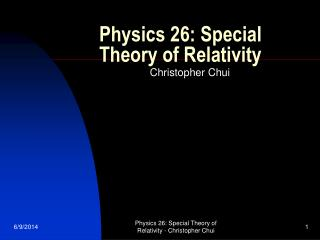DownloadDownload PresentationPhysics 26: Special Theory of Relativity

# Physics 26: Special Theory of Relativity

Télécharger la présentation## Physics 26: Special Theory of Relativity

- - - - - - - - - - - - - - - - - - - - - - - - - - - E N D - - - - - - - - - - - - - - - - - - - - - - - - - - -
##### Presentation Transcript

1. Physics 26: Special Theory of Relativity Christopher Chui Physics 26: Special Theory of Relativity - Christopher Chui

2. Galilean-Newtonian Relativity • Inertial reference frames are reference frames in which Newton’s 1st law, the law of inertia, is valid • Relativity principle: the laws of physics are the same in all inertial reference frame • In classical mechanics, space and time are absolute • All inertial reference frames are equally valid • Michelson-Morley experiment did not detect ether • Special relativity includes 2 postulates: the relativity principle and the constancy of the speed of light: light propagates through empty space with a definite speed c independent of the speed of the source or observer Physics 26: Special Theory of Relativity - Christopher Chui

3. Simultaneity is Relative • 2 simultaneous events to 1 observer may not be simultaneous to a 2nd observertime is not absolute • Time-dilation: clocks moving relative to an observer are measured by that observer to run more slowly • Formula: t = to/[1-v2/c2]½ where to is at rest frame • Twin paradox: the traveling twin will age less • Length contraction: L = Lo [1-v2/c2]½ where Lo is at rest frame • 4-dimensional space-time: time as the extra dimension • Mass increase: m = mo/[1-v2/c2]½ where mo is at rest • KE = mc2 – moc2  E = mc2 = moc2 + KE Physics 26: Special Theory of Relativity - Christopher Chui

4. Relativistic Momentum • p = mv = mov/ [1-v2/c2]½ • Energy-momentum relation: E2 = p2c2 + mo2c4 • Units for p is eV/c • Units for m is eV/c2 • Relativistic addition of velocities:u=(v+u’)/ [1+vu’/c2] when v and u’ in the same direction. For opposite directions, u’ is negative u=(v-u’)/ [1-vu’/c2] • A more general theory gives the same results as a more restricted theory is called the correspondence principle Physics 26: Special Theory of Relativity - Christopher Chui